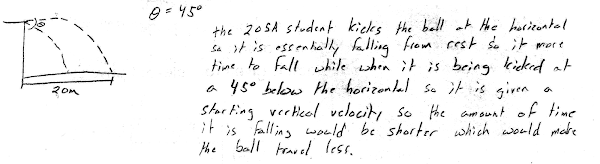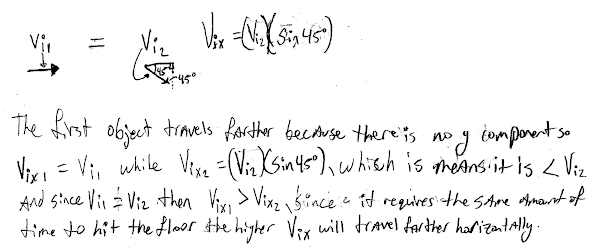## 20150105

### Physics final exam question: downwards-kicked soccer balls

Physics 205A Final Exam, fall semester 2014
Cuesta College, San Luis Obispo, CA

Cf. Giambattista/Richardson/Richardson, Physics, 2/e, Conceptual Questions 2.1, 2.2, 3.9A Physics 205A student kicks a soccer ball horizontally with a certain speed off the edge of a cliff, and observes it landing on the ground below at a distance of 20 m from the edge. If the student kicks the soccer ball off the edge of this cliff with the same speed, but at angle of 45° below the horizontal, discuss why it will land on the ground below at a distance shorter than 20 m. Explain your reasoning using the properties of projectile motion.

• p:
Correct. The ball kicked at a downwards angle will travel a shorter horizontal displacement Δx = vx⋅Δt because:
1. it has a slower horizontal velocity component vx;
2. and it has a shorter elapsed time Δt to travel before it hits the ground.
• r:
Nearly correct, but includes minor math errors. One of the two points (1)-(2) correct, other is problematic/incomplete.
• t:
Nearly correct, but approach has conceptual errors, and/or major/compounded math errors. Only one of the two points (1)-(2) correct, other is missing, or both are problematic.
• v:
Implementation of right ideas, but in an inconsistent, incomplete, or unorganized manner. Some garbled attempt at finding velocity components, and applying kinematic equations of constant (zero) acceleration.
• x:
Implementation of ideas, but credit given for effort rather than merit.
• y:
Irrelevant discussion/effectively blank. This may include restating what must be proved.
• z:
Blank.
Sections 70854, 70855, 73320
Exam code: finalm34T
p: 7 students
r: 2 students
t: 20 students
v: 11 students
x: 14 students
y: 8 students
z: 0 students

A sample "p" response (from student 0169):A sample "t" response (from student 0123), neglecting the difference in horizontal velocities:Another sample "t" response (from student 1107), neglecting the difference in elapsed times: